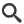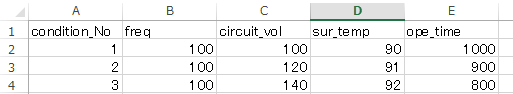:
Hz]
:
[V](peak to peak)
:
[V]nF
=
nF
nF
V
=
V
V
=
=
=
V
=
V
V
A
=
A
A
=
=
=
=
=
=
=<<>>
V(p-p) V(r.m.s.)

<<>>
[s] [ms] [us]<<>>
V(p-p) V(r.m.s.)kset/Mkbcs/M[nF]

[KHz]

[V(p-p)]

[A(r.m.s.)]

[℃]

[mm]

[mm]

[Hr]

[ppm]

[nF]

[V]

[V(p-p)]

[A(r.m.s.)]

[nF]

[mm2]